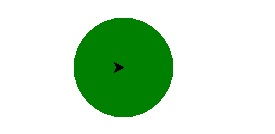# Python Online Quiz

Following quiz provides Multiple Choice Questions (MCQs) related to Python. You will have to read all the given answers and click over the correct answer. If you are not sure about the answer then you can check the answer using Show Answer button. You can use Next Quiz button to check new set of questions in the quiz.Q 1 - What is output for −

a = ['he', 'she', 'we']

' '.join(a)

A - ['heshewe']

B - 'heshewe'

C - ['he she we']

D - 'he she we'

### Explanation

The method join() takes list of string as input and returns string as output. It removes ', ' and add the given string with join to the list.

Q 2 - Which is invalid in python for z = 5 ?

A - z = z++

B - z = ++z

C - z += 1

D - z -= 1

### Explanation

z = z++ is not valid in python, it is not a legal expression. It results in syntax error.

Q 3 - Select the option for following code −

```s = 0
for d in range(0, 5, 0.1):
… s += d
… print(s)
```

A - Syntax Error

B - Type Error

C - Runtime Error

D - Both b & c

### Explanation

we will get type error during runtime as float object cannot be interpreted as integer. Here 0.1 is the float value.

### Explanation

since there is no such element in the set, so key error is raised.

Q 5 - Find the output of the code?

```def f(a, b = 1, c = 2):
print('a is: ',a, 'b is: ', b, 'c is: ', c)
f(2, c = 2)
f(c = 100, a = 110)
```

A - a is: 2 b is: 1 c is: 2

a is: 110 b is: 1 c is: 100

B - a is: 2 b is: 2 c is: 2

a is: 110 b is: 2 c is: 100

C - a is: 0 b is: 2 c is: 2

a is: 110 b is: 0 c is: 100

D - a is: 110 b is: 0 c is: 100

a is: 110 b is: 0 c is: 100

### Explanation

Value of Arguments is passed to the parameters in order of their sequence.

Q 6 - What is the output of the following code?

```class P:
def __init__(self):
self.__x=100
self.y=200
def print(self):
print(self.__x, self.y)
class C(P):
def __init__(self):
super().__init__()
self.__x=300
self.y=400
d = C()
d.print()
```

A - 300 400

B - 100 400

C - 100 200

D - 300 200

### Explanation

In the above code x is a private variable declared in the class P. Thus value of x cannot be changed in the class C which inherits class P. But y is not a private variable thus its value can be changed.

Q 7 - Which of the function among will return 4 on the set s = {3, 4, 1, 2}?

A - Sum(s)

B - Len(s)

C - Max(s)

D - Four(s)

### Explanation

len(s) returns the length of the set and max(s) returns the maximum value in the set.

Q 8 - Which event among them is fired when the right mouse button is released?

A - <ButtonReleased>

B - <ButtonPressed>

C - <ButtonReleased-3>

D - <ButtonPressed-3>

### Explanation

It is the default way to do this kind of work.

Q 10 - Select the code for the following output?A - Turtle.circle(20 , ''green '')

B - Turtle.circle(''green'')

C - Turtle.dot(20 , ''green'')

D - Turtle.dot(''green'')